Successfully reported this slideshow.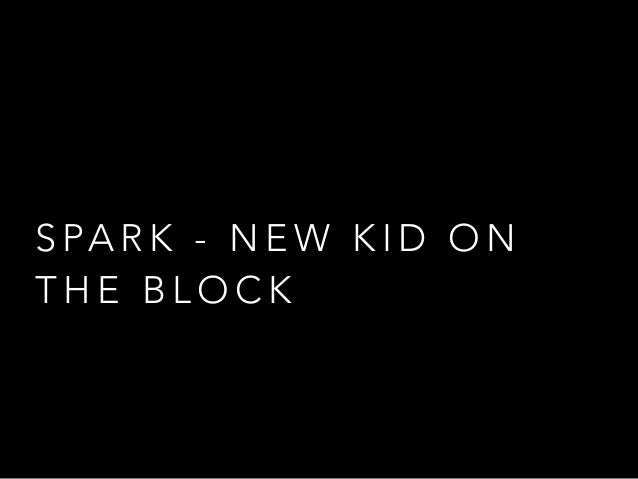Upcoming SlideShare
×

of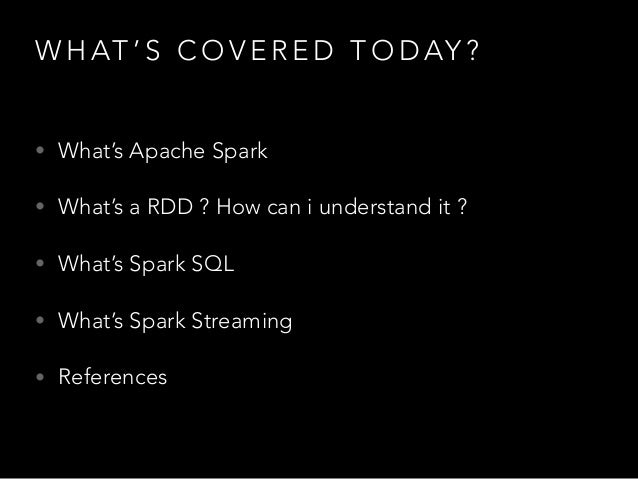4

Share

# Toying with spark

My talk for the Scala meetup at PayPal's Singapore office.
The intention is to focus on 3 things:
(a) two common functions in Apache Spark "aggregate" and "cogroup"
(b) Spark SQL
(c) Spark Streaming
The umbrella event is http://www.meetup.com/Singapore-Scala-Programmers/events/219613576/

See all

### Toying with spark

1. 1. S PA R K - N E W K I D O N T H E B L O C K
2. 2. A B O U T M E … • I designed Bamboo (HP’s Big Data Analytics Platform) • I write software (mostly with Scala but leaning towards Haskell recently …) • I like translating seq to parallel algorithms mostly using CUDA / OpenCL; embedded assembly is an EVIL thing. • I wrote 2 books • OpenCL Parallel Programming Development Cookbook • Developing an Akka Edge
3. 3. W H AT ’ S C O V E R E D T O D AY ? • What’s Apache Spark • What’s a RDD ? How can i understand it ? • What’s Spark SQL • What’s Spark Streaming • References
4. 4. W H AT ’ S A PA C H E S PA R K • As a beginner’s guide, you can refer to Tsai Li Ming’s talk. • API model abstracts • how to extract data from 3rd party s/w (via JDBC, Cassandra, HBase) • how to extract-compute data (via GraphX, MLLib, SparkSQL) • how to store data (data connectors to “local”, “hdfs”, “s3”
5. 5. R E S I L I E N T D I S T R I B U T E D D ATA S E T S • Apache Spark works on data broken into chunks • These chunks are called RDDs • RDDs are chained into a lineage graph => a graph that identifies relationships. • RDDs can be queried, grouped, transformed in a coarse grained manner to a fine grained manner.
6. 6. • A RDD has a lifecycle: • reification • lazy-compute/lazy re-compute • destruction • RDD’s lifecycle is managed by the system unless … • A program commands the RDD to persist() or unpersist() which affects the lazy computation. R E S I L I E N T D I S T R I B U T E D D ATA S E T S
7. 7. “ A G G R E G AT E ” I N S PA R K > val data = sc.parallelize( (1 to 4) toList,2) > data.aggregate(0) > .. (math.max(_, _), > .. ( _ + _ )) > ….. > result = 6 def aggregate(zerovalue: U) (fbinary: (U, T) => U, fagg: (U, U) => U): U
8. 8. H O W “ A G G R E G AT E ” W O R K S I N S PA R K e1 RDD fagg fbinary e2 e3 e4 zerovalue res1 fbinary res2 fagg final result caveat: partition-sensitive algorithm should work correctly regardless of partitions
9. 9. “ C O G R O U P ” I N S PA R K > val x = sc.parallelize(List(1, 2, 1, 3), 1) > val y = x.map((_, "y")) > val z = x.map((_, "z")) > y.cogroup(z).collect res72: Array[(Int, (Iterable[String], Iterable[String]))] = Array((1, (Array(y, y),Array(z, z))), (3,(Array(y),Array(z))), (2, (Array(y),Array(z)))) def cogroup[W1, W2, W3] (other1: RDD[(K, W1)], other2: RDD[(K, W2)], other3: RDD[(K, W3)], numPartitions: Int): RDD[(K, (Iterable[V], Iterable[W1], Iterable[W2], Iterable[W3]))]
10. 10. H O W “ C O G R O U P ” W O R K S I N S PA R K RDDx (k1,va) (k2,vb) (k1,vc) (k3,vd) (k1,ve) (k1,vf) (k2,vg) (k1,vh) RDDy RDDx.cogroup(RDDy) =?
11. 11. H O W “ C O G R O U P ” W O R K S I N S PA R K Arraycombined Array[(k1,[va,vc,ve,vf,vh]), (k2,[vb,vg]), (k3,[vd])] RDDx.cogroup(RDDy) = *see below*
12. 12. “ C O G R O U P ” I N S PA R K • CoGroup works in both RDD and Spark Streams • the ability to combine multiple RDDs allows higher abstractions to be constructed • A Stream in Spark is just a list of (Time,RDD[U])
13. 13. W H AT ’ S S PA R K S Q L • Spark SQL is new, largely replaced Shark • Large scale queries (inline queries) to be embedded into a Spark program • Spark SQL supports Apache Hive, JSON, Parquet, RDD. • Spark SQL’s optimizer is clever! • Supports UDFs from Hive or Write your own !
14. 14. S PA R K S Q L J S O N S PA R K S Q L PA R Q U E TH I V E data sources R D D
15. 15. S PA R K S Q L ( A N E X A M P L E ) // import spark sql import org.apache.spark.sql.hive.HiveContext // create a spark sql hivecontext val sc = new SparkContext(…) val hiveCtx = new HiveContext(sc)
16. 16. S PA R K S Q L ( A N E X A M P L E ) // import spark sql import org.apache.spark.sql.hive.HiveContext // create a spark sql hivecontext val sc = new SparkContext(…) val hiveCtx = new HiveContext(sc) val input = hiveCtx.jsonFile(inputFile) input.registerTempTable(“tweets”)
17. 17. S PA R K S Q L ( A N E X A M P L E ) // import spark sql import org.apache.spark.sql.hive.HiveContext // create a spark sql hivecontext val sc = new SparkContext(…) val hiveCtx = new HiveContext(sc) val input = hiveCtx.jsonFile(inputFile) input.registerTempTable(“tweets”) val topTweets = hiveCtx.sql(“SELECT text, retweetCount FROM tweets ORDER BY retweetCount LIMIT 10”)
18. 18. S PA R K S Q L ( A N E X A M P L E ) // import spark sql import org.apache.spark.sql.hive.HiveContext // create a spark sql hivecontext val sc = new SparkContext(…) val hiveCtx = new HiveContext(sc) val input = hiveCtx.jsonFile(inputFile) input.registerTempTable(“tweets”) val topTweets = hiveCtx.sql(“SELECT text, retweetCount FROM tweets ORDER BY retweetCount LIMIT 10”) val topTweetContent = topTweets.map(row ⇒ row.getString(0))
19. 19. W H AT ’ S S PA R K S T R E A M I N G • Core component is a DStream • DStream is an abstract RDD whose basic components is a (key,value) pairs where key = Time, value = RDD. • Forward and backward queries are supported • Fault-Tolerance by check-pointing RDDs. • What you can do with RDDs, you can do with DStreams.
20. 20. S PA R K S T R E A M I N G ( Q U I C K E X A M P L E ) import org.apache.spark.streaming.StreamingContext import org.apache.spark.streaming.StreamingContext._ import org.apache.spark.streaming.dstream.DStream import org.apache.spark.streaming.Duration // Create a StreamingContext with a 1-second batch size from a SparkConf val ssc = new StreamingContext(conf, Seconds(1))
21. 21. S PA R K S T R E A M I N G ( Q U I C K E X A M P L E ) import org.apache.spark.streaming.StreamingContext import org.apache.spark.streaming.StreamingContext._ import org.apache.spark.streaming.dstream.DStream import org.apache.spark.streaming.Duration // Create a StreamingContext with a 1-second batch size from a SparkConf val ssc = new StreamingContext(conf, Seconds(1))  // Create a DStream using data received after connecting to // port 7777 on the local machine val lines = ssc.socketTextStream("localhost", 7777)  // Filter our DStream for lines with "error"  val errorLines = lines.filter(_.contains("error"))  // Print out the lines with errors  errorLines.print()
22. 22. S PA R K S T R E A M I N G ( Q U I C K E X A M P L E ) import org.apache.spark.streaming.StreamingContext import org.apache.spark.streaming.StreamingContext._ import org.apache.spark.streaming.dstream.DStream import org.apache.spark.streaming.Duration // Create a StreamingContext with a 1-second batch size from a SparkConf val ssc = new StreamingContext(conf, Seconds(1))  // Create a DStream using data received after connecting to // port 7777 on the local machine val lines = ssc.socketTextStream("localhost", 7777)  // Filter our DStream for lines with "error"  val errorLines = lines.filter(_.contains("error"))  // Print out the lines with errors  errorLines.print() // Start our streaming context and wait for it to "finish" ssc.start()  // Wait for the job to finish ssc.awaitTermination()
23. 23. A D S T R E A M L O O K S L I K E … t1 to t2 t2 to t3 t3 to t4 timestart DStream
24. 24. A D S T R E A M C A N H AV E T R A N S F O R M AT I O N S O N T H E M ! t1 to t2 timestart DStream(s) t1 to t2 data-1 data-2 f transformation on the fly!
25. 25. S PA R K S T R E A M T R A N S F O R M AT I O N t1 to t2t2 to t3 timestart DStream(s) t1 to t2t2 to t3 data-1 data-2 f f data output in batches
26. 26. S PA R K S T R E A M T R A N S F O R M AT I O N t3 to t4 timestart DStream(s) t3 to t4 data-1 data-2 f t1 to t2t2 to t3 t1 to t2t2 to t3 f fff
27. 27. S TAT E F U L S PA R K S T R E A M T R A N S F O R M AT I O N t3 to t4 timestart DStream(s) t3 to t4 data-1 data-2 f t1 to t2t2 to t3 t1 to t2t2 to t3 f fff
28. 28. H O W D O E S S PA R K S T R E A M I N G H A N D L E FA U LT S ? • As before, check-point is the key to fault-tolerance (especially in stateful-dstream transformations) • Programs can recover from check-points => no need to restart all over again. • You can use “monit” to restart Spark jobs or pass the Spark flag “- - supervise” to the job config a.k.a driver fault tolerance
29. 29. • All incoming data to workers replicated • In-house RDDs follow the lineage graph to recover • The above is known as worker fault tolerance. • Receivers fault tolerance is largely dependent on whether data sources can re-send lost data • Streams guarantee exactly-once semantics; caveat: multiple writes can occur to the HDFS (app specific logic needs to handle) H O W D O E S S PA R K S T R E A M I N G H A N D L E FA U LT S ?
30. 30. R E F E R E N C E S • Books: • “Learning Spark: Lightning Fast Big Data ANlaytics” • “Advanced Analytics with Spark: Patterns for Learning from Data At Scale” • “Fast Data Processing with Spark” • “Machine Learning with Spark” • Berkeley Data Bootcamp • Introduction to Big Data with Apache Spark • Kien Dang’s introduction to Spark and R using Naive Bayes (click here) • Spark Streaming with Scala and Akka (click here)
31. 31. T H E E N D Q U E S T I O N S ? T W I T T E R : @ R AY M O N D TAY B L G I T H U B : @ R AY G I T
•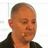#### bunkertor

Mar. 18, 2015
•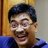Mar. 15, 2015
•#### dimitrisli

Feb. 26, 2015
•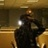#### lamida

Feb. 24, 2015

Total views

1,811

On Slideshare

0

From embeds

0

Number of embeds

168

9

Shares

0